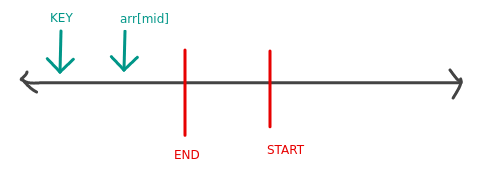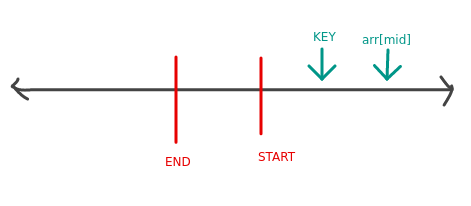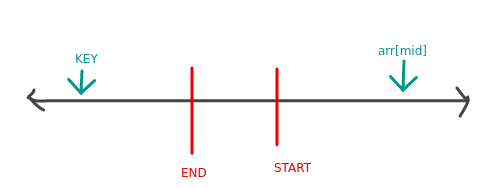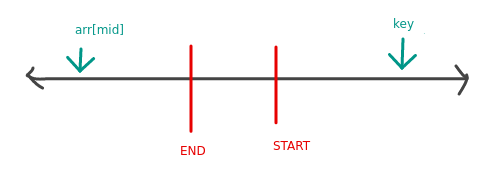# Searching in a rotated sorted array

Given a sorted array with ` n ` elements which is rotated at some point. There is a number ` key ` which needs to be searched in this array. Write a program which searches for this ` key ` in the array. The worst case time complexity of this solution should not exceed ` O(logn) `.

#### Example Test Cases

##### Sample Test Case 1

Array: ` 5, 6, 9, 0, 1, 2, 3, 4 `
Key: ` 6 `
Expected Output: ` 1 `
Explanation: Since ` 1 ` is the index of ` 6 `

#### Solution

Searching in a sorted array is a classic binary search problem and can be done in ` O(logn) ` time. Since this array is rotated along some point, normal binary search cannot be applied to it.
However this problem can be solved by modifying the binary search (without affecting it’s time complexity) by making the following observations.

• Since the array was sorted (before rotation), we can think of this array as concatenation of two separate sorted arrays. for example array ` 5, 6, 9, 0, 1, 2, 3, 4 ` can be thought of as two concatenation of ` 5, 6, 9 ` and ` 0, 1, 2, 3, 4 `

• Since, the 2 parts of the above array are themselves sorted, we can apply normal binary search on these parts. The only problem is that we don’t know the length of each of these two sorted parts.

• The 1st element of the whole array combined will always be greater than the last element. Let us call the 1st element as ` start ` and the last element as ` end `. So it means that ` start > end `.

Now, when we apply binary search to the problem either of these 4 cases can happen:

• Case 1 : Both the ` arr[mid] ` and the ` key ` are less than the ` end `. It means they are in the same half and we can apply normal binary search.• Case 2 : Both the ` arr[mid] ` and the ` key ` are greater than ` start `. It means they are in the same half and we can apply normal binary search.• Case 3 : The ` key ` is less than ` end ` but ` arr[mid] ` is greater than start. It means in this case, we need to change direction (to get ` mid ` in the same half as ` key ` ) before applying normal binary search.So in this case we will do ` lo = mid + 1 `

• Case 4 : The ` key ` is greater than ` end ` but ` arr[mid] ` is less than start. It means in this case, we need to change direction (to get ` mid ` in the same half as ` key ` ) before applying normal binary search.So in this case we will do ` hi = mid - 1 `

Therefore, to solve this problem we will start by applying normal binary search and then depending on which case we find, we will either apply normal binary search or we will try to switch direction and try to either go to the right half or to the left half.
See the code below for implementation

#### Implementation

```#include <iostream>
#include <vector>
using namespace std;

// Used to check which case we are in and give appropriate direction.
// 0 means Do normal binary search
int getDirection(vector<int>& arr, int mid, int key) {
int start = arr;
int end = arr[arr.size() - 1];
if (arr[mid] <= end && key <= end) {
return (0);
}
else if (arr[mid] >= start && key >= start) {
return (0);
}
else if (arr[mid] >= start && key < start) {
return 1;
}
else {
return -1;
}
}

int main() {
vector<int> arr = {5, 6, 9, 0, 1, 2, 3, 4 };
int key = 6;
int lo = 0, hi = arr.size() - 1;
int mid;
int ans = -1;
while (lo <= hi) {
mid = lo + (hi - lo)/2;
if (arr[mid] == key) {
ans = mid;
break;
}
else {
int direction = getDirection(arr, mid, key);
// Case 3
if (direction > 0) {
lo = mid + 1;
}
// Case 4
else if (direction < 0) {
hi = mid - 1;
}
// Case 1 and 2 (applying normal binary search)
else {
if (arr[mid] < key) {
lo = mid + 1;
}
else {
hi = mid - 1;
}
}
}
}

if (ans == -1) {
cout << "Key " << key << " not found\n";
}
else {
cout << "Key " << key << " found at " << ans << " position\n";
}
return 0;
}```

Scroll to Top

### Full Stack Integrated Bootcamp Free Trial

• Please enter a number from 7000000000 to 9999999999.